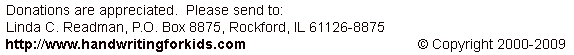Addition: Level 2: Exercise 4 (no carryovers)

Enter your answers in the boxes then click on SUBMIT:

 6+3 5+4 7+1 2+4 8+0 7+0 1+2 3+5 6+1 7+2 3+1 0+0 4+1 3+3 1+5 5+2 3+4 1+1 9+0 2+2 4+4 2+7 5+0 2+6 0+4 0+2 8+1 2+3 4+5 1+0